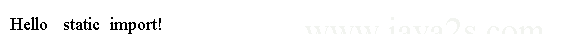Java Object Oriented Design - Java import

An import declaration is used to import any type into a compilation unit.

Import declarations appear just after the package declaration and before the first type declaration.

There are two types of import declarations:

• Single-type import declaration
• Import-on-demand declaration

Single-Type Import Declaration

A single-type import declaration is used to import a single type from a package. It is of the form.

```import <fully qualified name of a type>;
```

The following import declaration imports the Dog class from the com.java2s package:

```import com.java2s.Dog;
```

A single-type import declaration imports only one type(a class) from a package.

To import more than one type from a package, use a separate import declaration for each type.

The following import declarations import ClassOne from pkg1 package, ClassTwo and ClassThree from pkg2 package, and ClassFour from pkg3 package:

```import pkg1.ClassOne;
import pkg2.ClassTwo;
import pkg2.ClassThree;
import pkg3.ClassFour;
```

The following code uses fully qualified name of the Dog class.

```public  class  Main{
public static  void  main(String[]  args)  {
com.java2s.Dog jack;  // Uses  full qualified name for the   Dog  class
}
}
```

The following code shows how to use a single-type-import declaration to import the com.java2s.Dog class to its simple name.

The modified Main class declaration is as follows:

```import com.java2s.Dog; // Import   the   Dog  class

public  class  Main {
public static  void  main(String[]  args)  {
Dog  jack; // Use simple name of  the   Dog  class
}
}
```

When the compiler comes across the simple name of the Dog class in the statement, like

```Dog  jack;
```

it goes through all import declarations to resolve the simple name to a fully qualified name.

When it tries to resolve the simple name Dog, it finds the import declaration, import com.java2s.Dog, which imports the Dog class.

It assumes that you intended to use the com.java2s.Dog class when you used the simple name Dog in the above statement.

The compiler replaces the above statement with the following statement:

```com.java2s.Dog jack;
```

Import declarations let you use the simple name of a type in your code, thus making your code more readable.

When you compile your code, the compiler replaces the simple name of a type with its fully qualified name.

It uses import declarations for converting simple names of the types to their fully qualified names.

Import-on-Demand Declaration

An import-on-demand declaration imports multiple types from a package using one import declaration.

The syntax for an import-on-demand declaration is

```import <package name>.*;
```

Here, the package name is followed by a dot and an asterisk (*).

For example, the following import-on-demand declaration imports all types from com.java2s package:

```import com.java2s.*;
```

You can rewrite the code for the Main class using an import-on-demand declaration.

```import com.java2s.*;

public  class  Main {
public static  void  main(String[]  args)  {
Dog  jack; // Use simple name of  the   Dog  class
}
}
```

Static Import Declarations

A static import declaration imports static members (variables/methods) of a type into a compilation unit.

A static import declaration comes in two flavors: single-static import and static-import-on-demand.

A single-static import declaration imports one static member from a type. A static-import-on-demand declaration imports all static members of a type.

The general syntax of static import declaration is as follows:

Single-static-import declaration:

```import static <package  name>.<type name>.<static member name>;
```

Static-import-on-demand declaration:

```import static <package  name>>.<type  name>.*;
```

System is a class in java.lang package that has a static variable named out.

When you use System.out, we are referring to that static variable out of the System class.

We can use a static import declaration to import the out static variable from the System class as follows:

```import static  java.lang.System.out;
```

The following code imports the out static variable of the System class.

```import static  java.lang.System.out;

public class  StaticImportTest  {
public static  void  main(String[]  args)  {
out.println("Hello static  import!");
}
}
```

The code above generates the following result.The Math class in the java.lang package has many utility constants and static methods.

For example, it has a class variable named PI.

To use any of the static variables or methods of the Math class, we will need to qualify them with the class name Math.

For example, you would refer to the PI static variable as Math.PI and the sqrt() method as Math.sqrt().

We can import all static members of the Math class using the following static-import- on-demand declaration:

```import static  java.lang.Math.*;
```

Example

The following code demonstrates using the Math class by importing its static members.

```import static java.lang.Math.PI;
import static java.lang.Math.sqrt;
import static java.lang.System.out;
/*from w  w w  .  ja v  a  2  s  . c o  m*/
public class Main {
public static void main(String[] args) {

out.println("Value of  PI  is: " + PI);
out.println("Area of  circle: " + area);
out.println("Square root of  2.0: " + sqrt(2.0));
}
}
```

The code above generates the following result.The following are some important rules about static import declaration.

• If two static members with the same simple name are imported, one using single-static import declaration and other using static-import-on-demand declaration, the one imported using single-static import declaration takes precedence.
• Using single-static-import declaration to import two static members with the same simple name is not allowed.
• If a static member is imported using a single-static import declaration and there exists a static member in the same class with the same name, the static member in the class is used.